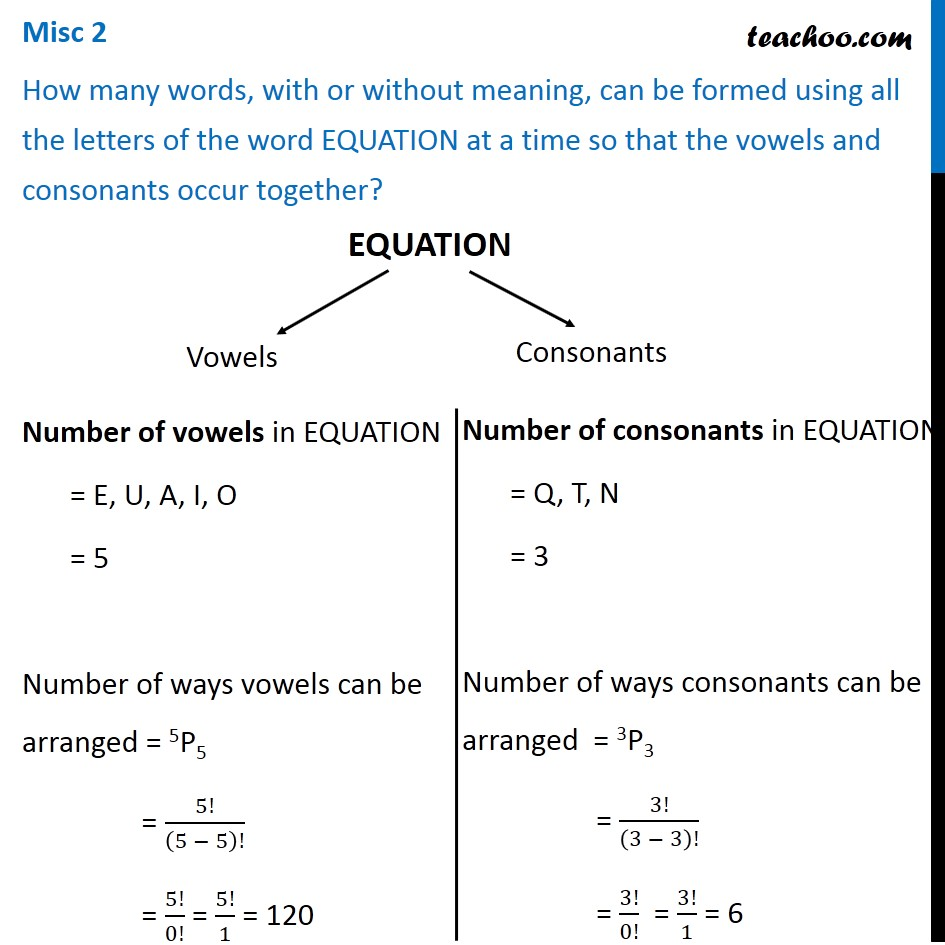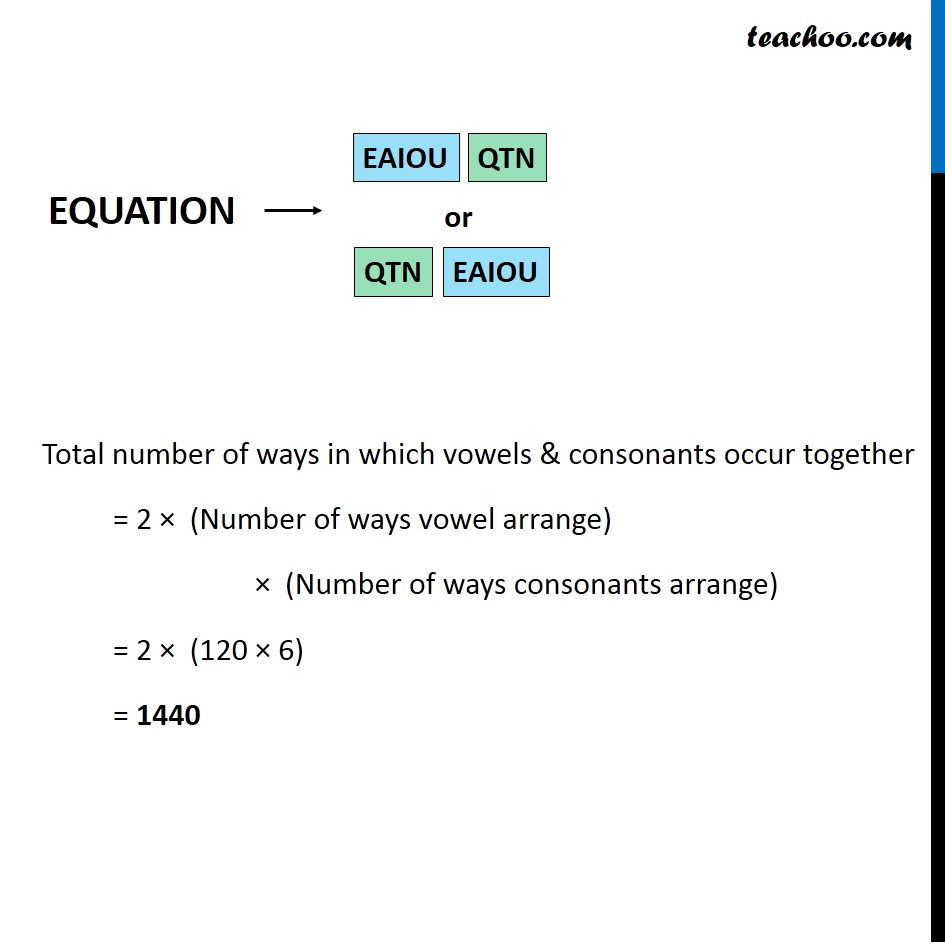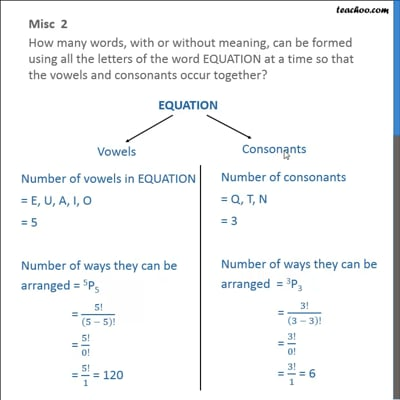Miscellaneous

Chapter 7 Class 11 Permutations and Combinations (Term 2)
Serial order wiseThis video is only available for Teachoo black users

### Transcript

Misc 2 How many words, with or without meaning, can be formed using all the letters of the word EQUATION at a time so that the vowels and consonants occur together? Misc 2 How many words, with or without meaning, can be formed using all the letters of the word EQUATION at a time so that the vowels and consonants occur together? Number of vowels in EQUATION = E, U, A, I, O = 5 Number of ways vowels can be arranged = 5P5 = 5!/(5 − 5)! = 5!/0! = 5!/1 = 120 Number of consonants in EQUATION = Q, T, N = 3 Number of ways consonants can be arranged = 3P3 = 3!/(3 − 3)! = 3!/0! = 3!/1 = 6 Total number of ways in which vowels & consonants occur together = 2 × (Number of ways vowel arrange) × (Number of ways consonants arrange) = 2 × (120 × 6) = 1440